# Decimals

A decimal point separates whole numbers from numbers with value less than 1.

## Example: 3.232

 Thousands Hundreds Tens Units Tenths Hundredths Thousandths 3 2 3 2

The number 3, which is to the right, is a whole number and the numbers to the left are as follows:

• Tenths = 2/10
• Hundredths = 3/100
• Thousandths = 2/1000

# More Examples!

## Example 1

6.22

Six is a whole number (6 units) and .22 is less than one
2/10 + 2/100 = 22/100.

 Th H T U 1/10 1/100 1/1000 6 2 2

## Example 2

56.134

56 is a whole number (5 tens and 6 units) and .134 is less than one
1/10 + 3/100 + 4/1000 = 134/1000.

 Th H T U 1/10 1/100 1/1000 5 6 1 3 4

## Example 3

734.356

734 is a whole number (7 hundreds,3 tens and 4 units) and .356 is less than one
3/10 + 5/100 + 6/1000 = 356/1000.

 Th H T U 1/10 1/100 1/1000 7 3 4 3 5 6

We have to align the decimal points, before we add up.

U 1/10
2. 4 +
5. 1
7. 5
U 1/10 1/100
4. 2 9
2. 3 8 +
6. 6 7
1
T U 1/10 1/100
1 6. 5 2
3 5. 2 4 +
5 1. 7 6
1

# Subtraction

Just like addition, we have to align the decimal points.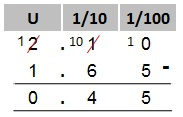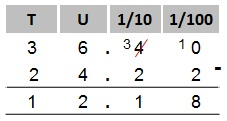# Multiplication

Example 1: 2.5 x 3 = ?

1. Remove the decimal point
and multiply as normal.

Note! 2.5 has only one number after the decimal
point.

 2 5 x 3 7 5 1

2. Add a decimal point after the 7.
Hence, the answer has one number after the
decimal point.

Example 2: 4.2 x 2.1=?

1. Remove the decimal points and multiply
as normal.

Note! 4.2 has one number after the decimal
point and 2.1 has one number after the decimal
point 1+1=2.

 4 2 x 2 1 4 2 8 4 0 8 8 2

2. The answer should have two numbers
after the decimal point. Hence, add the
decimal point after the 8.

# Multiply by 10

If we multiply by 10 we move the decimal point one place to the right.

## Example 1

3.5 × 10= ?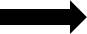## Example 2

4.678 × 10= ?## Example 3

45.43 × 10= ?# Multiply by 100

If we multiply by 100 we move the decimal point two places to the right.

## Example 1

4.56 x 100= ?## Example 2

13.23 x 100= ?## Example 3

3.384 x 100= ?# Multiply by 1000

If we multiply by 1000 we move the decimal point three places to the right.

## Example 1

0.64 x 1000= ?## Example 2

1.230 x 1000= ?## Example 3

7.254 x 1000= ?# Division

Divide as normal but the decimal point for the answer should be aligned to the decimal point inside the division bracket.

## Example 1

Divide 5.5 by 5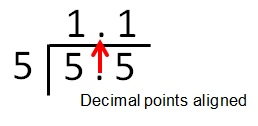## Example 2

Divide 12.3 by 3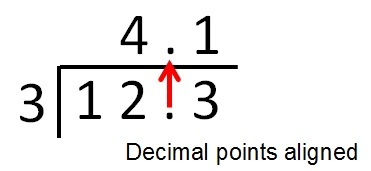In the above division we divided decimal numbers by whole numbers. Now we are going to divide decimal numbers by decimal numbers.

## Example 1

7.5 ÷ 2.5 = ?

1. Multiply both the numbers by 10 to make it easier for us to divide.

7.5 x 10 = 75
2.5 x 10 = 25

2. Divide 75 by 25Dividing 75 by 25 is the same as dividing 7.5 by 2.5. Therefore the answer is 3

.

## Example 2

1.4412 ÷ 0.12=?

1. Multiply both the numbers by 100 to make it easier for us to divide.

1.4412 x 100 = 144.12
0.12 x 100 = 12

2. Divide 144.12 by 12.
It helps if you know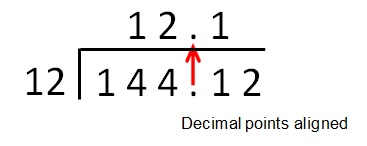Dividing 144.12 by 12 is the same as dividing 1.4412 by 0.12 Therefore the answer is 12.1.

# Divide by 10

If we divide by 10 we move the decimal point one place to the left.

## Example 1

44 ÷ 10= ?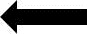## Example 2

67.42 ÷ 10= ?## Example 3

4.54 x 10= ?# Divide by 100

If we divide by 100 we move the decimal point two places to the left.

## Example 1

236.2 ÷ 100= ?## Example 2

12.34 ÷ 100= ?## Example 3

3.45 ÷ 100= ?# Divide by 1000

If we divide by 1000 we move the decimal point three places to the left.

## Example 1

435.2 ÷ 1000= ?## Example 2

12382.5 ÷= ?## Example 3

5325.34 ÷= ?# Recurring Decimal Number

A recurring decimal number is a number that is continously repeated. For example dividing 2 by 3 will give us a continous repeated decimal number 6.
2/3 = 0.6666666666666

0.66666666666 can be shortened to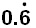# Decimals to Percentages

Multiply the decimal number by 100 for example 0.5 x 100= 50%

0.546 x 100 = 54.6%

0.035 x 100 = 3.5%

To convert the percentages back to decimals divide by 100.

# Fractions to Decimals

2/5 means 2 divide by 5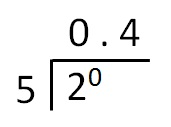2/5 = 0.4

# Decimals to Fractions

0.4 means 4/10

.
 Th H T U 1/10 1/100 1/1000 4

We can say 4/10 equals 2/5 by dividing the top and bottom numbers by 2.

0.75 means 7/10 + 5/100 = 75/100

 Th H T U 1/10 1/100 1/1000 7 5

We can say 75/100 = 3/4 by dividing the top and bottom number by 25.

# Whole Numbers

Whole numbers can be converted into fractions. For example we know 0.4 means 4/10 so 1.4 would mean 10/10 + 4/10 = 14/10.

## More Examples!

2.4 in fractions means 20/10 + 4/10 = 24/10

3.6 in fractions means 30/10 + 6/10 = 36/10

1.75 in fractions means 100/100 + 75/100 = 175/100

2.75 in fraction means 200/100 + 75/100 = 275/100

Now try the Exercises!